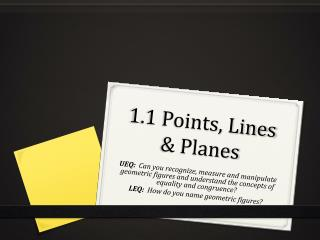DownloadDownload Presentation1.1 Points, Lines & Planes

# 1.1 Points, Lines & Planes

Télécharger la présentation## 1.1 Points, Lines & Planes

- - - - - - - - - - - - - - - - - - - - - - - - - - - E N D - - - - - - - - - - - - - - - - - - - - - - - - - - -
##### Presentation Transcript

1. 1.1 Points, Lines & Planes UEQ: Can you recognize, measure and manipulate geometric figures and understand the concepts of equality and congruence? LEQ: How do you name geometric figures?

2. 1.1 Points, Lines & Planes Undefined Terms Terms that can only be explained using examples: Point  is a location having neither shape or size Point A (capital) Line  a bunch of points defined by min 2 points line m (lowercase) line AB line AB line AB Plane  a flat surface made of points extending infinitely in all directions Plane K (capital italic) Plane ABC (order not *)

3. Points, Lines & Planes Point Line Plane

4. In the figure below name: Finite Plane • 4 Points: J K L M • Line: JK, JL, KL, JL, a • Plane: B, JKL, KLM, KLJ • … is a plane that has boundaries, it does not extend indefinitely. Example: a piece of paper

5. Intersecting geometric figures Intersection of two or more figures is the set of points they have in common. Two (or more) lines AB & CD can intersect at a Point X Intersection 2 Lines D A X B C

6. Intersecting geometric figures Two planes, W & Z can intersect and form a line AB Intersection 2 Planes

7. Intersecting geometric figures A plane X intersects with a line m at point Z Intersection Plane & Line m Z X

8. Intersecting geometric figures Which geometric figure is formed when 3 planes intersect? Answer: Either a point or a line Intersection 3 Planes

9. What are the following: Spear Arrow Baton Golf ball Piece of paper Wall Edge of roof Vector X-axis Dumbbell Handrail

10. 1.1 Points, Lines & Planes UEQ: Can you recognize, measure and manipulate geometric figures and understand the concepts of equality and congruence? LEQ: How do you name geometric figures?